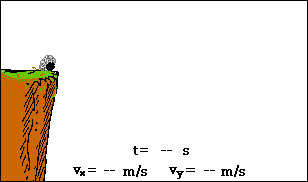## Non-Horizontally Launched Projectiles

Imagine a cannonball being launched at an angle from a cannon atop a very high cliff. Imagine as well that the cannonball does not encounter a significant amount of air resistance. What would be the path of the cannonball and how could the motion of the cannonball be described? The animation below depicts such a situation. The path of the cannonball is shown; additionally, the horizontal and vertical velocity components are represented by arrows in the animation.As can be seen from the above animation, the cannonball follows a parabolic path. As the cannonball rises towards its peak, it undergoes a downward acceleration. An upwardly moving cannonball which is slowing down is said to be undergoing a downward acceleration. As the cannonball falls, it still undergoes a downward acceleration. A downward moving cannonball which is gaining speed is said to have a downward acceleration. In the animation, the downward acceleration is depicted by a change in the vertical component of velocity. This downward acceleration is caused by the downward force of gravity which acts upon the ball. If the ball's motion could be approximated as projectile motion (that is, if the influence of air resistance could be assumed negligible), then there would be no horizontal acceleration. In the absence of horizontal forces, there would be a constant velocity in the horizontal direction. In the animation, this is depicted by the fact that the horizontal velocity component remains the same size throughout the entire motion of the cannonball.

Many would insist that there is a horizontal force acting upon the cannonball since it is moving horizontally. This is simply not the case. The horizontal motion of the cannonball is the result of its own inertia. When launched from the cannon, the cannonball already possessed a horizontal motion. The cannonball will maintain this state of horizontal motion unless acted upon by a horizontal force. An object in motion will continue in motion with the same speed and in the same direction ... (Newton's first law). Remind yourself continuously: forces are not required for an object to be moving. Once in motion, the presence of forces will only serve to accelerate such objects.

For more information on physical descriptions of motion, visit The Physics Classroom Tutorial. Detailed information is available there on the following topics:

Acceleration of Gravity

Direction of Acceleration

Projectiles

Characteristics of a Projectile's Trajectory

Newton's First Law of Motion

Inertia and the State of Motion